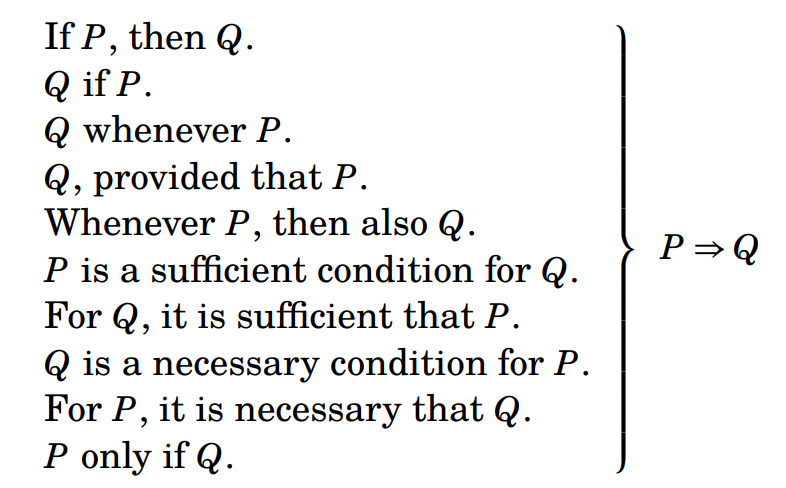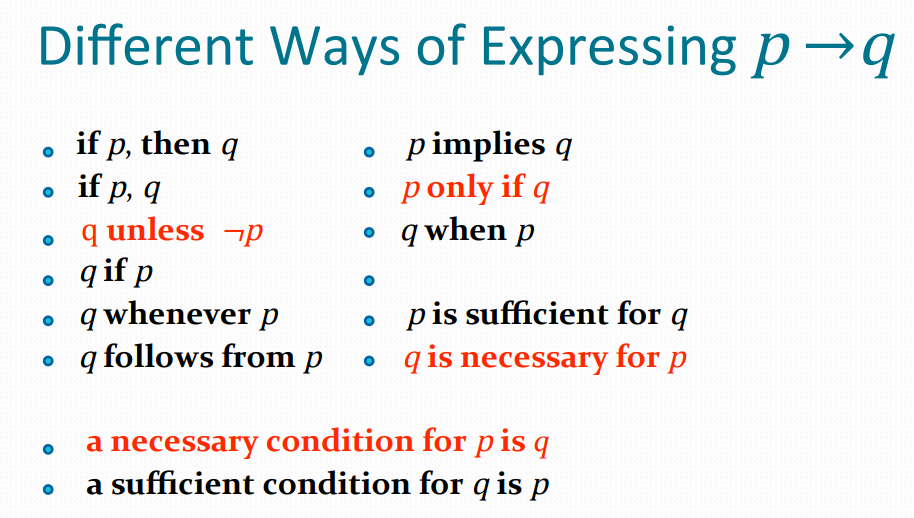575 views

Let $p$ be the statement “Maria learns discrete mathematics” and $q$ the statement “Maria will find a good job.” Which of the following English Statement expresses the statement $p \rightarrow q$ ?
1. “If Maria learns discrete mathematics, then she will find a good job.”
2. “Maria will find a good job provided that she learns discrete mathematics.”
3. “Maria will learn discrete mathematics provided that she finds a good job.”
4. “For Maria to get a good job, it is sufficient for her to learn discrete mathematics.”option A : “if Maria learns discrete mathematics , then she will find a good job”
by

1 vote